### NCERT Solutions For Class 11 Math Chapter – 6 Exercise – 6.1

NCERT Solutions For Class 11 Math  Chapter – 6 Exercise – 6.11. Solve 24x < 100, when

(i) x is a natural number.

(ii) x is an integer.

Solution:

(i) Given that 24x < 100

Now we have to divide the inequality by 24 then we get x < 25/6

Now when x is a natural integer then

It is clear that the only natural number less than 25/6 are 1, 2, 3, 4.

Thus, 1, 2, 3, 4 will be the solution of the given inequality when x is a natural number.

Hence

{1, 2, 3, 4} is the solution set.

(ii) Given that 24x < 100

Now we have to divide the inequality by 24 then we get x < 25/6

now when x is an integer then

It is clear that the integer number less than 25/6 are…-1, 0, 1, 2, 3, 4.

Thus, solution of 24 x < 100 are…,-1, 0, 1, 2, 3, 4, when x is an integer.

Hence {…, -1, 0, 1, 2, 3, 4} is the solution set.

2. Solve – 12x > 30, when

(i) x is a natural number.

(ii) x is an integer.

Solution:

(i) Given that, – 12x > 30

Now by dividing the inequality by -12 on both sides we get, x < -5/2

When x is a natural integer then

It is clear that there is no natural number less than -2/5 because -5/2 is a negative number and natural numbers are positive numbers.

Therefore there would be no solution of the given inequality when x is a natural number.

(ii) Given that, – 12x > 30

Now by dividing the inequality by -12 on both sides we get, x < -5/2

When x is an integer then

It is clear that the integer number less than -5/2 are…, -5, -4, – 3

Thus, solution of – 12x > 30 is …,-5, -4, -3, when x is an integer.

Therefore the solution set is {…, -5, -4, -3}

3. Solve 5x – 3 < 7, when

(i) x is an integer

(ii) x is a real number

Solution:

(i) Given that, 5x – 3 < 7

Now by adding 3 both side we get,

5x – 3 + 3 < 7 + 3

Above inequality becomes

5x < 10

Again by dividing both sides by 5 we get,

5x/5 < 10/5

x < 2

When x is an integer then

It is clear that that the integer number less than 2 are…, -2, -1, 0, 1.

Thus, solution of 5x – 3 < 7 is …,-2, -1, 0, 1, when x is an integer.

Therefore the solution set is {…, -2, -1, 0, 1}

(ii) Given that, 5x – 3 < 7

Now by adding 3 both side we get,

5x – 3 + 3 < 7 + 3

Above inequality becomes

5x < 10

Again by dividing both sides by 5 we get,

5x/5 < 10/5

x < 2

When x is a real number then

It is clear that the solutions of 5x – 3 < 7 will be given by x < 2 which states that all the real numbers that are less than 2.

Hence the solution set is x ∈ (-∞, 2)

4. Solve 3x + 8 >2, when

(i) x is an integer.

(ii) x is a real number.

Solution:

(i) Given that, 3x + 8 > 2

Now by subtracting 8 from both sides we get,

3x + 8 – 8 > 2 – 8

The above inequality becomes,

3x > – 6

Again by dividing both sides by 3 we get,

3x/3 > -6/3

Hence x > -2

When x is an integer then

It is clear that the integer number greater than -2 are -1, 0, 1, 2,…

Thus, solution of 3x + 8 > 2is -1, 0, 1, 2,… when x is an integer.

Hence the solution set is {-1, 0, 1, 2,…}

(ii) Given that, 3x + 8 > 2

Now by subtracting 8 from both sides we get,

3x + 8 – 8 > 2 – 8

The above inequality becomes,

3x > – 6

Again by dividing both sides by 3 we get,

3x/3 > -6/3

Hence x > -2

When x is a real number.

It is clear that the solutions of 3x + 8 >2 will be given by x > -2 which states that all the real numbers that are greater than -2.

Therefore the solution set is x ∈ (-2, ∞)

Solve the inequalities in Exercises 5 to 16 for real x.

5. 4x + 3 < 5x + 7

Solution:

Given that, 4x + 3 < 5x + 7

Now by subtracting 7 from both the sides, we get

4x + 3 – 7 < 5x + 7 – 7

The above inequality becomes,

4x – 4 < 5x

Again by subtracting 4x from both the sides,

4x – 4 – 4x < 5x – 4x

x > – 4

∴The solutions of the given inequality are defined by all the real numbers greater than -4.

Required solution set is (-4, ∞)

6. 3x – 7 > 5x – 1

Solution:

Given that,

3x – 7 > 5x – 1

Now by adding 7 to both the sides, we get

3x – 7 +7 > 5x – 1 + 7

3x > 5x + 6

Again by subtracting 5x from both the sides,

3x – 5x > 5x + 6 – 5x

-2x > 6

Dividing both sides by -2 to simplify we get

-2x/-2 < 6/-2

x < -3

∴ The solutions of the given inequality are defined by all the real numbers less than -3.

Hence the required solution set is (-∞, -3)

7. 3(x – 1) ≤ 2 (x – 3)

Solution:

Given that, 3(x – 1) ≤ 2 (x – 3)

By multiplying above inequality can be written as

3x – 3 ≤ 2x – 6

Now by adding 3 to both the sides, we get

3x – 3+ 3 ≤ 2x – 6+ 3

3x ≤ 2x – 3

Again by subtracting 2x from both the sides,

3x – 2x ≤ 2x – 3 – 2x

x ≤ -3

Therefore the solutions of the given inequality are defined by all the real numbers less than or equal to -3.

Hence the required solution set is (-∞, -3]

8. 3 (2 – x) ≥ 2 (1 – x)

Solution:

Given that, 3 (2 – x) ≥ 2 (1 – x)

By multiplying we get

6 – 3x ≥ 2 – 2x

Now by adding 2x to both the sides,

6 – 3x + 2x ≥ 2 – 2x + 2x

6 – x ≥ 2

Again by subtracting 6 from both the sides, we get

6 – x – 6 ≥ 2 – 6

– x ≥ – 4

Multiplying throughout inequality by negative sign we get

x ≤ 4

∴ The solutions of the given inequality are defined by all the real numbers greater than or equal to 4.

Hence the required solution set is (- ∞, 4]

9. x + x/2 + x/3 < 11

Solution: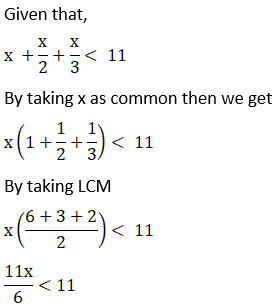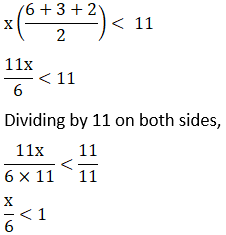x < 6

The solutions of the given inequality are defined by all the real numbers less than 6.

Hence the solution set is (-∞, 6)

10. x/3 > x/2 + 1

Solution: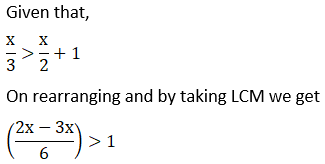– x/6 > 1

– x > 6

x < – 6

∴ The solutions of the given inequality are defined by all the real numbers less than – 6.

Hence the required solution set is (-∞, -6)

11. 3(x – 2)/5 ≤ 5 (2 – x)/3

Solution:

Given that,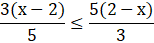Now by cross – multiplying the denominators, we get

9(x- 2) ≤ 25 (2 – x)

9x – 18 ≤ 50 – 25x

Now adding 25x both the sides,

9x – 18 + 25x ≤ 50 – 25x + 25x

34x – 18 ≤ 50

34x – 18 + 18 ≤ 50 + 18

34x ≤ 68

Dividing both sides by 34,

34x/34 ≤ 68/34

x ≤ 2

The solutions of the given inequality are defined by all the real numbers less than or equal to 2.

Required solution set is (-∞, 2]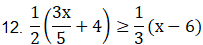Solution: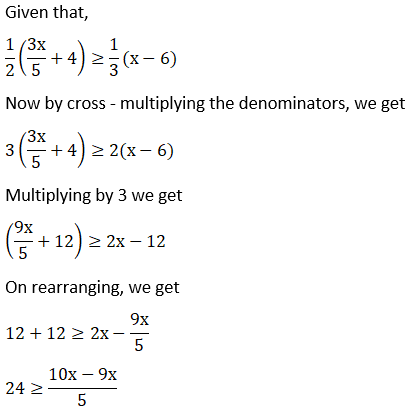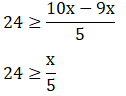120 ≥ x

∴ The solutions of the given inequality are defined by all the real numbers less than or equal to 120.

Thus, (-∞, 120] is the required solution set.

13. 2 (2x + 3) – 10 < 6 (x – 2)

Solution:

Given that,

2 (2x + 3) – 10 < 6 (x – 2)

By multiplying we get

4x + 6 – 10 < 6x – 12

On simplifying we get

4x – 4 < 6x – 12

– 4 + 12 < 6x – 4x

8 < 2x

4 < x

∴ The solutions of the given inequality are defined by all the real numbers greater than or equal to 4.

Hence the required solution set is (4, -∞)

14. 37 – (3x + 5) ≥ 9x – 8 (x – 3)

Solution:

Given that, 37 – (3x + 5) ≥ 9x – 8 (x – 3)

On simplifying we get

= 37 – 3x – 5 ≥ 9x – 8x + 24

= 32 – 3x ≥ x + 24

On rearranging

= 32 – 24 ≥ x + 3x

= 8 ≥ 4x

= 2 ≥ x

All the real numbers of x which are less than or equal to 2 are the solutions of the given inequality

Hence, (-∞, 2] will be the solution for the given inequality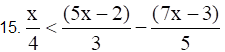Solution: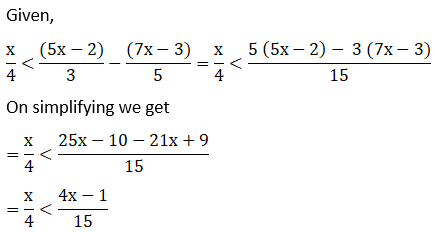= 15x < 4 (4x – 1)

= 15x < 16x – 4

= 4 < x

All the real numbers of x which are greater than 4 are the solutions of the given inequality

Hence, (4, ∞) will be the solution for the given inequality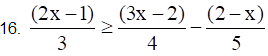Solution: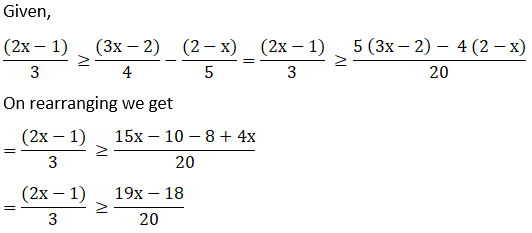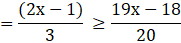= 20 (2x – 1) ≥ 3 (19x – 18)

= 40x – 20 ≥ 57x – 54

= – 20 + 54 ≥ 57x – 40x

= 34 ≥ 17x

= 2 ≥ x

∴ All the real numbers of x which are less than or equal to 2 are the solutions of the given inequality

Hence, (-∞, 2] will be the solution for the given inequality

Solve the inequalities in Exercises 17 to 20 and show the graph of the solution in each case on number line.

17. 3x – 2 < 2x + 1

Solution:

Given,

3x – 2 < 2x + 1

Solving the given inequality, we get

3x – 2 < 2x + 1

= 3x – 2x < 1 + 2

= x < 3

Now, the graphical representation of the solution is as follows: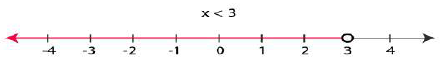18. 5x – 3 ≥ 3x – 5

Solution:

We have,

5x – 3 ≥ 3x – 5

Solving the given inequality, we get

5x – 3 ≥ 3x – 5

On rearranging we get

= 5x – 3x ≥ -5 + 3

On simplifying

= 2x ≥ -2

Now divide by 2 on both sides we get

= x ≥ -1

The graphical representation of the solution is as follows: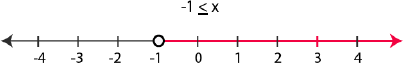19. 3 (1 – x) < 2 (x + 4)

Solution:

Given,

3 (1 – x) < 2 (x + 4)

Solving the given inequality, we get

3 (1 – x) < 2 (x + 4)

Multiplying we get

= 3 – 3x < 2x + 8

On rearranging we get

= 3 – 8 < 2x + 3x

= – 5 < 5x

Now by dividing 5 on both sides we get

-5/5 < 5x/5

= – 1 < x

Now, the graphical representation of the solution is as follows: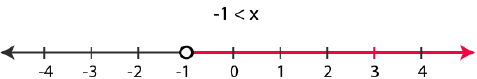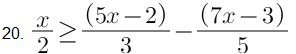Solution: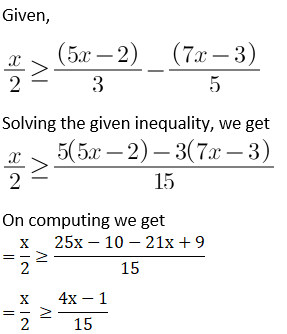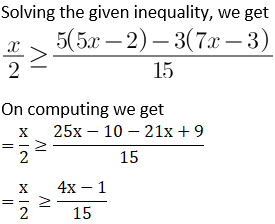On computing we get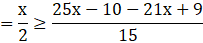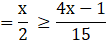= 15x ≥ 2 (4x – 1)

= 15x ≥ 8x -2

= 15x -8x ≥ 8x -2 -8x

= 7x ≥ -2

= x ≥ -2/7

Now, the graphical representation of the solution is as follows: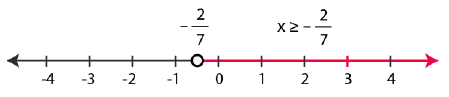21. Ravi obtained 70 and 75 marks in first two unit test. Find the minimum marks he should get in the third test to have an average of at least 60 marks.

Solution:

Let us assume, x be the marks obtained by Ravi in his third unit test

According to question, the entire students should have an average of at least 60 marks

(70 + 75 + x)/3 ≥ 60

= 145 + x ≥ 180

= x ≥ 180 – 145

= x ≥ 35

Hence, all the students must obtain 35 marks in order to have an average of at least 60 marks

22. To receive Grade ‘A’ in a course, one must obtain an average of 90 marks or more in five examinations (each of 100 marks). If Sunita’s marks in first four examinations are 87, 92, 94 and 95, find minimum marks that Sunita must obtain in fifth examination to get grade ‘A’ in the course.

Solution:

Let us assume Sunita scored x marks in her fifth examination

Now, according to the question in order to receive A grade in the course she must have to obtain average 90 marks or more in her five examinations

(87 + 92 + 94 + 95 + x)/5 ≥ 90

= (368 + x)/5 ≥ 90

= 368 + x ≥ 450

= x ≥ 450 – 368

= x ≥ 82

Hence, she must have to obtain 82 or more marks in her fifth examination

23. Find all pairs of consecutive odd positive integers both of which are smaller than 10 such that their sum is more than 11.

Solution:

Let us assume x be the smaller of the two consecutive odd positive integers

∴ Other integer is = x + 2

It is also given in the question that, both the integers are smaller than 10

∴ x + 2 < 10

x < 8 … (i)

Also, it is given in the question that sum off two integers is more than 11

∴ x + (x + 2) > 11

2x + 2 > 11

x > 9/2

x > 4.5 … (ii)

Thus, from (i) and (ii) we have x is an odd integer and it can take values 5 and 7

Hence, possible pairs are (5, 7) and (7, 9)

24. Find all pairs of consecutive even positive integers, both of which are larger than 5 such that their sum is less than 23.Solution:

Let us assume x be the smaller of the two consecutive even positive integers

∴ Other integer = x + 2

It is also given in the question that, both the integers are larger than 5

∴ x > 5   ….(i)

Also, it is given in the question that the sum of two integers is less than 23.

∴ x + (x + 2) < 23

2x + 2 < 23

x < 21/2

x < 10.5   …. (ii)

Thus, from (i) and (ii) we have x is an even number and it can take values 6, 8 and 10

Hence, possible pairs are (6, 8), (8, 10) and (10, 12).

25. The longest side of a triangle is 3 times the shortest side and the third side is 2 cm shorter than the longest side. If the perimeter of the triangle is at least 61 cm, find the minimum length of the shortest side.

Solution:

Let us assume the length of the shortest side of the triangle be x cm

∴ According to the question, length of the longest side = 3x cm

And, length of third side = (3x – 2) cm

As, the least perimeter of the triangle = 61 cm

Thus, x + 3x + (3x – 2) cm ≥ 61 cm

= 7x – 2 ≥ 61

= 7x ≥ 63

Now divide by 7 we get

= 7x/7 ≥ 63/7

= x ≥ 9

Hence, the minimum length of the shortest side will be 9 cm

26. A man wants to cut three lengths from a single piece of board of length 91cm.
The second length is to be 3cm longer than the shortest and the third length is to be twice as long as the shortest. What are the possible lengths of the shortest board if the third piece is to be at least 5cm longer than the second?

Solution:

Let us assume the length of the shortest piece be x cm

∴ According to the question, length of the second piece = (x + 3) cm

And, length of third piece = 2x cm

As all the three lengths are to be cut from a single piece of board having a length of 91 cm

∴ x + (x + 3) + 2x ≤ 91 cm

= 4x + 3 ≤ 91

= 4x ≤ 88

= 4x/4 ≤ 88/4

= x ≤ 22 … (i)

Also, it is given in the question that, the third piece is at least 5 cm longer than the second piece

∴ 2x ≥ (x+3) + 5

2x ≥ x + 8

x ≥ 8 … (ii)

Thus, from equation (i) and (ii) we have:

8 ≤ x ≤ 22

Hence, it is clear that the length of the shortest board is greater than or equal to 8 cm and less than or equal to 22 cm.